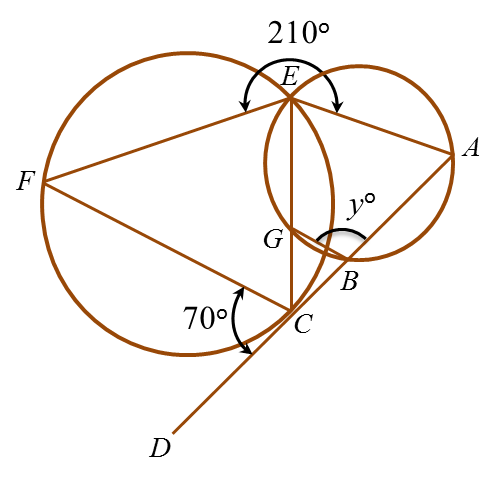# 6.7.3 Angles and Tangents of Circles, PT3 Focus Practice

Question 7:In figure above, ABCD is a tangent to the circle CEF at point C. EGC is a straight line. The value of y is

Solution:
$\begin{array}{l}\angle CEF=\angle DCF={70}^{\circ }\\ \angle AEG+{70}^{\circ }+{210}^{\circ }={360}^{\circ }\\ \angle AEG={80}^{\circ }\\ \\ \text{In cyclic quadrilateral}ABGE,\\ \angle ABG+\angle AEG={180}^{\circ }\\ {y}^{\circ }+{80}^{\circ }={180}^{\circ }\\ y=100\end{array}$

 
  
Question 8:In figure above, ABC is a tangent to the circle centre O at B. AED is a straight line.
 
Find the value of y.
  

  Solution:
 
ABO = 90o
 
BOE = 2 × 40o = 80o
 
 
AEO = 360– ∠ABO  – ∠BOE – 35o
 
= 360– 90o – 80o – 35= 155o
 
yo+ ∠AEO = 180
 
yo+ 155o = 180o
 
y= 180o  – 155o
 
y o = 25